# LBP原理介绍以及算法实现

http://blog.csdn.net/xidianzhimeng/article/details/19634573

LBPLocal Binary Pattern，局部二值模式）是一种用来描述图像局部纹理特征的算子；它具有旋转不变性和灰度不变性等显著的优点。它是首先由T. Ojala, M.Pietikäinen, D. Harwood 1994年提出，用于纹理特征提取。而且，提取的特征是图像的局部的纹理特征；

1LBP特征的描述

原始的LBP算子定义为在3*3的窗口内，以窗口中心像素为阈值，将相邻的8个像素的灰度值与其进行比较，若周围像素值大于中心像素值，则该像素点的位置被标记为1，否则为0。这样，3*3邻域内的8个点经比较可产生8位二进制数（通常转换为十进制数即LBP码，共256种），即得到该窗口中心像素点的LBP值，并用这个值来反映该区域的纹理信息。如下图所示：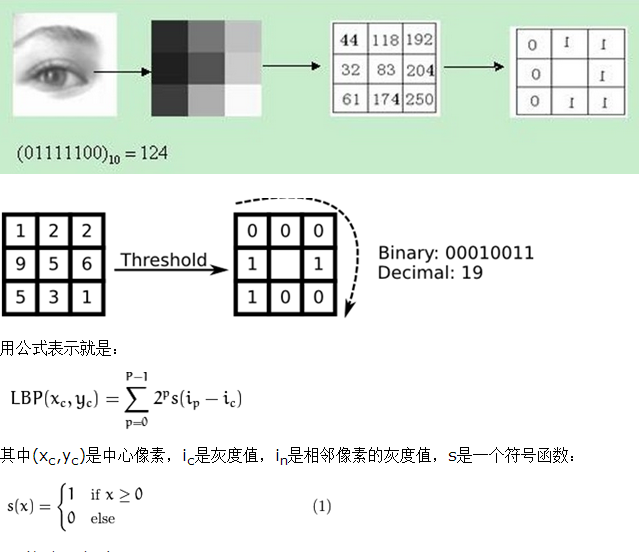LBP的改进版本：

原始的LBP提出后，研究人员不断对其提出了各种改进和优化。

1）圆形LBP算子：

基本的 LBP 算子的最大缺陷在于它只覆盖了一个固定半径范围内的小区域，这显然不能满足不同尺寸和频率纹理的需要。为了适应不同尺度的纹理特征，并达到灰度和旋转不变性的要求，Ojala 等对 LBP 算子进行了改进，将 3×邻域扩展到任意邻域，并用圆形邻域代替了正方形邻域，改进后的 LBP 算子允许在半径为 R 的圆形邻域内有任意多个像素点。从而得到了诸如半径为R的圆形区域内含有P个采样点的LBP算子；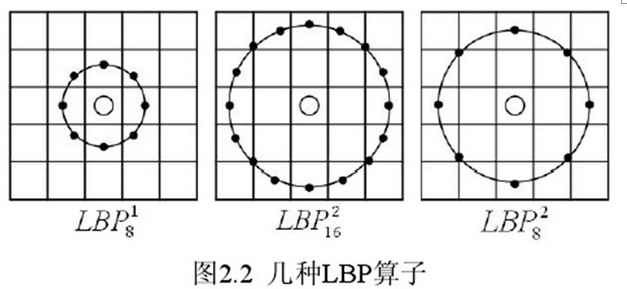2LBP旋转不变模式

从 LBP 的定义可以看出，LBP 算子是灰度不变的，但却不是旋转不变的。图像的旋转就会得到不同的 LBP值。

Maenpaa等人又将 LBP 算子进行了扩展，提出了具有旋转不变性的 LBP 算子，即不断旋转圆形邻域得到一系列初始定义的 LBP 值，取其最小值作为该邻域的 LBP 值。

图 2.5 给出了求取旋转不变的 LBP 的过程示意图，图中算子下方的数字表示该算子对应的 LBP 值，图中所示的 8  LBP模式，经过旋转不变的处理，最终得到的具有旋转不变性的 LBP 值为 15。也就是说，图中的 8  LBP 模式对应的旋转不变的 LBP 模式都是00001111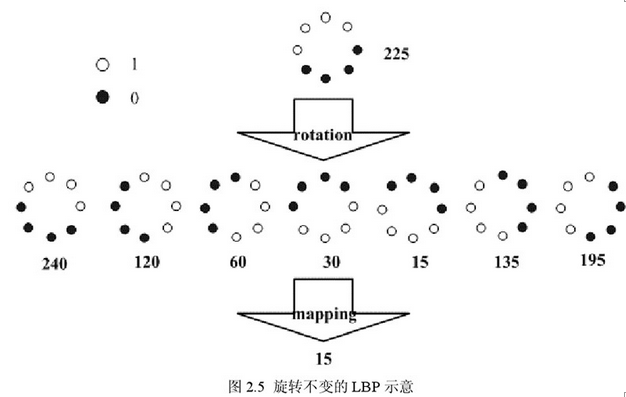3LBP等价模式

一个LBP算子可以产生不同的二进制模式，对于半径为R的圆形区域内含有P个采样点的LBP算子将会产生P2 种模式。很显然，随着邻域集内采样点数的增加，二进制模式的种类是急剧增加的。例如：5×5邻域内20个采样点，有2201,048,576种二进制模式。如此多的二值模式无论对于纹理的提取还是对于纹理的识别、分类及信息的存取都是不利的。同时，过多的模式种类对于纹理的表达是不利的。例如，将LBP算子用于纹理分类或人脸识别时，常采用LBP模式的统计直方图来表达图像的信息，而较多的模式种类将使得数据量过大，且直方图过于稀疏。因此，需要对原始的LBP模式进行降维，使得数据量减少的情况下能最好的代表图像的信息。

为了解决二进制模式过多的问题，提高统计性，Ojala提出了采用一种“等价模式”（Uniform Pattern）来对LBP算子的模式种类进行降维。Ojala等认为，在实际图像中，绝大多数LBP模式最多只包含两次从10或从01的跳变。因此，Ojala将“等价模式”定义为：当某个LBP所对应的循环二进制数从01或从10最多有两次跳变时，该LBP所对应的二进制就称为一个等价模式类。000000000次跳变），00000111（只含一次从01的跳变），10001111（先由1跳到0，再由0跳到1，共两次跳变）都是等价模式类。除等价模式类以外的模式都归为另一类，称为混合模式类，例如10010111（共四次跳变）（这是我的个人理解，不知道对不对）。

通过这样的改进，二进制模式的种类大大减少，而不会丢失任何信息。模式数量由原来的2P种减少为 P ( P-1)+2种，其中P表示邻域集内的采样点数。对于3×3邻域内8个采样点来说，二进制模式由原始的256种减少为58种，这使得特征向量的维数更少，并且可以减少高频噪声带来的影响。

2LBP特征用于检测的原理

显而易见的是，上述提取的LBP算子在每个像素点都可以得到一个LBP“编码”，那么，对一幅图像（记录的是每个像素点的灰度值）提取其原始的LBP算子之后，得到的原始LBP特征依然是“一幅图片”（记录的是每个像素点的LBP值）。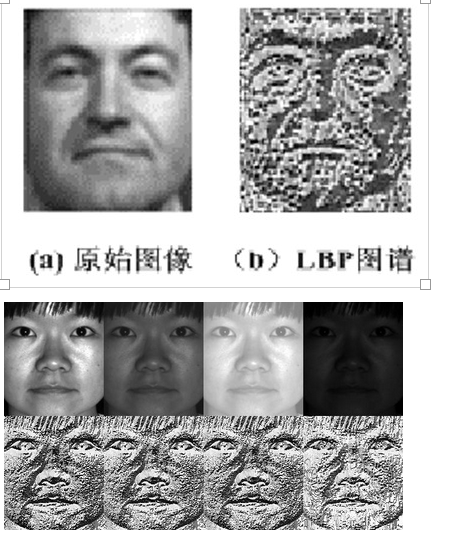LBP的应用中，如纹理分类、人脸分析等，一般都不将LBP图谱作为特征向量用于分类识别，而是采用LBP特征谱的统计直方图作为特征向量用于分类识别。

因为，从上面的分析我们可以看出，这个“特征”跟位置信息是紧密相关的。直接对两幅图片提取这种“特征”，并进行判别分析的话，会因为“位置没有对准”而产生很大的误差。后来，研究人员发现，可以将一幅图片划分为若干的子区域，对每个子区域内的每个像素点都提取LBP特征，然后，在每个子区域内建立LBP特征的统计直方图。如此一来，每个子区域，就可以用一个统计直方图来进行描述；整个图片就由若干个统计直方图组成；

例如：一幅100*100像素大小的图片，划分为10*10=100个子区域（可以通过多种方式来划分区域），每个子区域的大小为10*10像素；在每个子区域内的每个像素点，提取其LBP特征，然后，建立统计直方图；这样，这幅图片就有10*10个子区域，也就有了10*10个统计直方图，利用这10*10个统计直方图，就可以描述这幅图片了。之后，我们利用各种相似性度量函数，就可以判断两幅图像之间的相似性了；

3、对LBP特征向量进行提取的步骤

1）首先将检测窗口划分为16×16的小区域（cell）；

2）对于每个cell中的一个像素，将相邻的8个像素的灰度值与其进行比较，若周围像素值大于中心像素值，则该像素点的位置被标记为1，否则为0。这样，3*3邻域内的8个点经比较可产生8位二进制数，即得到该窗口中心像素点的LBP值；

3）然后计算每个cell的直方图，即每个数字（假定是十进制数LBP值）出现的频率；然后对该直方图进行归一化处理。

4）最后将得到的每个cell的统计直方图进行连接成为一个特征向量，也就是整幅图的LBP纹理特征向量；

Reference

4 opencv中如何提取LBP特征

（1） opencv1.0中LBP的实现-原始LBP特征

1. //基于旧版本的opencv的LBP算法opencv1.0
2. void LBP (IplImage *src,IplImage *dst)
3. {
4.     int tmp={0};
5.     CvScalar s;
6.
7.     IplImage * temp = cvCreateImage(cvGetSize(src), IPL_DEPTH_8U,1);
8.     uchar *data=(uchar*)src->imageData;
9.     int step=src->widthStep;  // 图像位宽
10.
11.     cout<<"step"<<step<<endl;
12.
13.     for (int i=1;i<src->height-1;i++)
14.       for(int j=1;j<src->width-1;j++)
15.       {
16.           int sum=0;
17.           if(data[(i-1)*step+j-1]>data[i*step+j])  // 左上角
18.             tmp=1;
19.
20.           if(data[i*step+(j-1)]>data[i*step+j])   // 上方
21.             tmp=1;
22.
23.           if(data[(i+1)*step+(j-1)]>data[i*step+j])  // 右上角
24.             tmp=1;
25.
26.           if (data[(i+1)*step+j]>data[i*step+j])  // 右侧
27.             tmp=1;
28.
29.           if (data[(i+1)*step+(j+1)]>data[i*step+j])  // 右下角
30.             tmp=1;
31.
32.           if(data[i*step+(j+1)]>data[i*step+j])  // 下方
33.             tmp=1;
34.
35.           if(data[(i-1)*step+(j+1)]>data[i*step+j])  // 左下角
36.             tmp=1;
37.
38.           if(data[(i-1)*step+j]>data[i*step+j])  // 左侧
39.             tmp=1;
40.
41.           //计算LBP编码
42.             s.val=(tmp*1+tmp*2+tmp*4+tmp*8+tmp*16+tmp*32+tmp*64+tmp*128);
43.             cvSet2D(dst,i,j,s);写入LBP图像
1.       }
（2）后期版本的LBP
a 圆形LBP算子
template <typename _Tp> static  // 模板函数，根据不同的原始数据类型得到不同的结果
inline void elbp_(InputArray _src, OutputArray _dst, int radius, int neighbors)
{
//get matrices
Mat src = _src.getMat();
// allocate memory for result因此不用在外部给_dst分配内存空间，输出数据类型都是int
Mat dst = _dst.getMat();
// zero
dst.setTo(0);
for(int n=0; n<neighbors; n++)
{
// sample points 获取当前采样点
float x = static_cast<float>(-radius) * sin(2.0*CV_PI*n/static_cast<float>(neighbors));
float y = static_cast<float>(radius) * cos(2.0*CV_PI*n/static_cast<float>(neighbors));
// relative indices 下取整和上取整
int fx = static_cast<int>(floor(x));  // 向下取整
int fy = static_cast<int>(floor(y));
int cx = static_cast<int>(ceil(x));   // 向上取整
int cy = static_cast<int>(ceil(y));
// fractional part 小数部分
float tx = x - fx;
float ty = y - fy;
// set interpolation weights 设置四个点的插值权重
float w1 = (1 - tx) * (1 - ty);
float w2 =      tx  * (1 - ty);
float w3 = (1 - tx) *      ty;
float w4 =      tx  *      ty;
// iterate through your data 循环处理图像数据
{
{
// calculate interpolated value 计算插值，t表示四个点的权重和
float t = w1*src.at<_Tp>(i+fy,j+fx) +
w2*src.at<_Tp>(i+fy,j+cx) +
w3*src.at<_Tp>(i+cy,j+fx) +
w4*src.at<_Tp>(i+cy,j+cx);
// floating point precision, so check some machine-dependent epsilon
//  std::numeric_limits<float>::epsilon()=1.192092896e-07F
// 当t>=src(i,j)的时候取1，并进行相应的移位
(std::abs(t-src.at<_Tp>(i,j)) < std::numeric_limits<float>::epsilon())) << n;
}
}
}
}

// 外部接口，根据不同的数据类型调用模板函数
static void elbp(InputArray src, OutputArray dst, int radius, int neighbors)
{
int type = src.type();
switch (type) {
case CV_8SC1:   elbp_<char>(src,dst, radius, neighbors); break;
case CV_8UC1:   elbp_<unsigned char>(src, dst, radius, neighbors); break;
case CV_16SC1:  elbp_<short>(src,dst, radius, neighbors); break;
case CV_16UC1:  elbp_<unsigned short>(src,dst, radius, neighbors); break;
case CV_32SC1:  elbp_<int>(src,dst, radius, neighbors); break;
case CV_32FC1:  elbp_<float>(src,dst, radius, neighbors); break;
case CV_64FC1:  elbp_<double>(src,dst, radius, neighbors); break;
default:
string error_msg = format("Using Circle Local Binary Patterns for feature extraction only works                                     on single-channel images (given %d). Please pass the image data as a grayscale image!", type);
CV_Error(CV_StsNotImplemented, error_msg);
break;
}
}
Mat cv::elbp(InputArray src, int radius, int neighbors) {
Mat dst;
return dst;
}
b 原始LBP算子
// 原始LBP算子只是计算8邻域内的局部二值模式
template <typename _Tp> static
void olbp_(InputArray _src, OutputArray _dst) {
// get matrices
Mat src = _src.getMat();
// allocate memory for result
_dst.create(src.rows-2, src.cols-2, CV_8UC1);
Mat dst = _dst.getMat();
// zero the result matrix
dst.setTo(0);
// calculate patterns
for(int i=1;i<src.rows-1;i++)
{
for(int j=1;j<src.cols-1;j++)
{
_Tp center = src.at<_Tp>(i,j);
unsigned char code = 0;
code |= (src.at<_Tp>(i-1,j-1) >= center) << 7;
code |= (src.at<_Tp>(i-1,j) >= center) << 6;
code |= (src.at<_Tp>(i-1,j+1) >= center) << 5;
code |= (src.at<_Tp>(i,j+1) >= center) << 4;
code |= (src.at<_Tp>(i+1,j+1) >= center) << 3;
code |= (src.at<_Tp>(i+1,j) >= center) << 2;
code |= (src.at<_Tp>(i+1,j-1) >= center) << 1;
code |= (src.at<_Tp>(i,j-1) >= center) << 0;
dst.at<unsigned char>(i-1,j-1) = code;
}
}
}
// 外部接口，根据不同的数据类型调用模板函数
void cv::olbp(InputArray src, OutputArray dst) {
switch (src.getMat().type()) {
case CV_8SC1:   olbp_<char>(src,dst); break;
case CV_8UC1:   olbp_<unsigned char>(src,dst); break;
case CV_16SC1:  olbp_<short>(src,dst); break;
case CV_16UC1:  olbp_<unsigned short>(src,dst); break;
case CV_32SC1:  olbp_<int>(src,dst); break;
case CV_32FC1:  olbp_<float>(src,dst); break;
case CV_64FC1:  olbp_<double>(src,dst); break;
default:
string error_msg = format("Using Original Local Binary Patterns for feature extraction only works                                     on single-channel images (given %d). Please pass the image data as a grayscale image!", type);
CV_Error(CV_StsNotImplemented, error_msg);
break;
}
}
Mat cv::olbp(InputArray src) {
Mat dst;
olbp(src, dst);
return dst;
}

1 Opencv1.0中原始LBP在处理相等的时候按照0进行处理，而在Opencv以后的版本中是按照1进行处理的
2 opencv1.0中输出LBP Map与源图像同等尺寸，而opencv以后的版本中原始LBP算子输出的结果比原始图像长宽上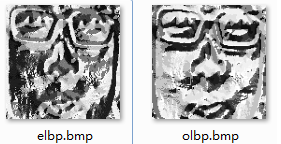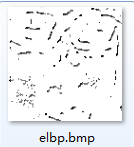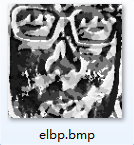(3) 计算整幅图像的LBPH
// 计算LBPM的空间直方图分布，得到一个一维向量
// src为LBPM是通过olbp或者elbp计算得到的
// numPatterns为计算LBP的模式数目，一般为2的幂
// grid_x和grid_y分别为每行或每列的block个数
// normed为是否进行归一化处理
static Mat spatial_histogram(InputArray _src, int numPatterns,
int grid_x, int grid_y, bool normed)
{
Mat src = _src.getMat();
// allocate memory for the spatial histogram为LBPH分配内存空间
Mat result = Mat::zeros(grid_x * grid_y, numPatterns, CV_32FC1);
// return matrix with zeros if no data was given，如果没有输入数据，返回的是0
if(src.empty())
return result.reshape(1,1);
// calculate LBP patch size block的尺寸
int width = src.cols/grid_x;
int height = src.rows/grid_y;
// initial result_row 初始化结果行
int resultRowIdx = 0;
// iterate through grid
for(int i = 0; i < grid_y; i++)
{
for(int j = 0; j < grid_x; j++)
{
// 获取指定区域
Mat src_cell = Mat(src, Range(i*height,(i+1)*height), Range(j*width,(j+1)*width));
// 计算指定区域的直方图
Mat cell_hist = histc(src_cell, 0, (numPatterns-1), true);
// copy to the result matrix 将计算得到的结果拷贝到每一行
Mat result_row = result.row(resultRowIdx);
cell_hist.reshape(1,1).convertTo(result_row, CV_32FC1);
// increase row count in result matrix
resultRowIdx++;
}
}
// return result as reshaped feature vector
return result.reshape(1,1);
}
（4） 从image到LBPH
int neighbor = 8;
Mat lbp_image = elbp(cv::cvarrToMat(m_refFaceImg), radius, neighbor); //robust performance
Mat query = spatial_histogram(
lbp_image,  /* lbp_image */
m_numPatterns, /* number of possible patterns可为 static_cast<int>(std::pow(2.0, static_cast<double>(_neighbors)))*/
m_grid_x,  /* grid size x */
m_grid_y,  /* grid size y */
true  /* normed histograms */
);03-06
04-01618
03-18431
04-023万+
01-175143
07-196979
01-059686
03-162869